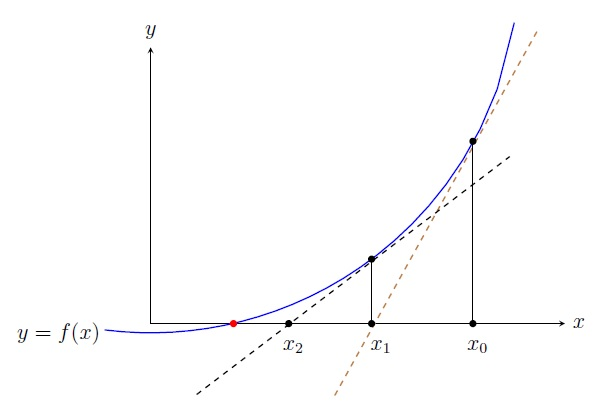Numerical Analysis Home

## Newton-Raphson Method

Suppose $$f$$ is a function with a unique root $$x^*$$ in $$[a,b]$$. Assume $$f''$$ is continuous in $$[a,b]$$. To find $$x^*$$, let $$x_0$$ be a "good" initial approximation (i.e., $$|x^*-x_0|^2 \approx 0$$) where $$f'(x_0)\neq 0$$. Using Taylor's Theorem on $$f$$ about $$x_0$$, we get $f(x)=f(x_0)+\frac{f'(x_0)}{1!}(x-x_0) +\frac{f''(\xi)}{2!}(x-x_0)^2,$ for some number $$\xi$$ (depends on $$x$$) between $$x_0$$ and $$x$$. Plugging $$x=x^*$$, we get \begin{align*} & 0=f(x^*)=f(x_0)+\frac{f'(x_0)}{1!}(x^*-x_0) +\frac{f''(\xi)}{2!}(x^*-x_0)^2\\ \implies & f(x_0)+\frac{f'(x_0)}{1!}(x^*-x_0) =-\frac{f''(\xi)}{2!}(x^*-x_0)^2 \approx 0 \;\;(\text{since }|x^*-x_0|^2\approx 0) \end{align*} Now solving for $$x^*$$, we get $x^*\approx x_0-\frac{f(x_0)}{f'(x_0)}.$ So $$x_1=x_0-\frac{f(x_0)}{f'(x_0)}$$ is an approximation to $$x^*$$. Using $$x_1$$, similarly we get $$x_2=x_1-\frac{f(x_1)}{f'(x_1)}$$. Continuing the process, we get a sequence $$\{x_n\}$$ to approximate $$x^*$$ where $\boxed{x_{n+1}=x_n-\frac{f(x_n)}{f'(x_n)},\; n=0,1,2,\ldots}$Observation. $$x_{n+1}$$ is the $$x$$-intercept of the tangent line to $$y=f(x)$$ at $$(x_n,f(x_n))$$.

The equation of the tangent line to $$y=f(x)$$ at $$(x_n,f(x_n))$$ is $y=f(x_n)+f'(x_n)(x-x_n).$ For the $$x$$-intercept, $$y=0$$. So $0=f(x_n)+f'(x_n)(x-x_n)\implies x=x_n-\frac{f(x_n)}{f'(x_n)}.$ Thus $$x_{n+1}$$ is the $$x$$-intercept of the tangent line to $$y=f(x)$$ at $$(x_n,f(x_n))$$.

Remark. We will show later that $$\{x_n\}$$ converges to $$x^*$$ for a good choice of an initial approximation $$x_0$$. If $$x_0$$ is far from $$x^*$$, then $$\{x_n\}$$ may not converge to $$x^*$$. For example, $$f(x)=x^2-5$$ has a unique root $$x^*=\sqrt{5}$$ in $$[-2,3]$$. But the Newton-Raphson Method constructs a sequence $$\{x_n\}$$ using $$x_0=-2$$ that converges to $$-\sqrt{5}\neq x^*$$.

Example. Do four iterations by the Newton-Raphson Method to approximate the root $$x^*$$ of $$f(x)=e^x-x-2$$ on the interval $$[0,2]$$ using $$x_0=1$$.

Solution. $$f(x)=e^x-x-2 \implies f'(x)=e^x-1$$ and $$f'(1)=e-1\neq 0$$. Thus $x_{n+1} =x_n-\frac{e^{x_n}-x_n-2}{e^{x_n}-1},\; n=0,1,2,\ldots$ $\begin{array}{|l|l|c|l|l|} \hline n & x_n & f(x_n) & f'(x_n) & x_{n+1}=x_n-\frac{f(x_n)}{f'(x_n)}\\ \hline 0 & 1 & -0.2817 & 1.7182 & 1.1639 \\ \hline 1 & 1.1639 & 0.0384 & 2.2023 & 1.1464 \\ \hline 2 & 1.1464 & 0.0004 & 2.1468 & 1.1462 \\ \hline 3 & 1.1462 & 0.0000 & 2.1462 & 1.1462 \\ \hline \end{array}$ Since $$|x_4-x_3|<10^{-4}$$, we can say that the root is $$x_4=1.1462$$ roughly correct to four decimal places (almost true as $$x^*=1.146193$$). Actually $$x_4$$ is correct to 12 decimal places! Note that this sequence converges to the root faster than that of other methods (why?).

Convergence: Suppose $$f$$ is a function with a simple root $$x^*$$ in $$[a,b]$$, i.e., $$f'(x^*)\neq 0$$. Assume $$f''$$ is continuous in $$[a,b]$$. Then there is a $$\delta>0$$ such that the sequence $$\{x_n\}$$ constructed by the Newton-Raphson Method converges to $$x^*$$ for any choice of the initial approximation $$x_0\in (x^*-\delta,x^*+\delta)$$.

Consider the following function $$g$$ on $$[a,b]$$: $g(x)=x-\frac{f(x)}{f'(x)}$ First note that $$x^*$$ is a fixed point of $$g$$ in $$[a,b]$$. Since $$f'(x^*)\neq 0$$, by the continuity of $$f'$$ in $$[a,b]$$, there is a $$\delta_1>0$$ such that $$f'(x)\neq 0$$ for all $$x\in [x^*-\delta_1,x^*+\delta_1]$$. So $$g$$ is continuous on $$[x^*-\delta_1,x^*+\delta_1]$$.

The convergence follows by that of the Fixed Point Iteration of $$g$$ on $$[x^*-\delta,x^*+\delta]$$ if we can show that there is a positive $$\delta<\delta_1$$ such that (i) $$x^*-\delta\leq g(x)\leq x^*+\delta$$ for all $$x\in [x^*-\delta,x^*+\delta]$$ and (ii) $$|g'(x)|<1$$ for all $$x\in(x^*-\delta,x^*+\delta)$$. To prove (ii), note that \begin{align*} & g'(x)=1-\frac{f'(x)f'(x)-f(x)f''(x)}{(f'(x))^2}=\frac{f(x)f''(x)}{(f'(x))^2}\\ \implies & g'(x^*)=\frac{f(x^*)f''(x^*)}{(f'(x^*))^2}=0\;\; (\text{since }f(x^*)=0,\;f'(x^*)\neq 0) \end{align*} Since $$g'(x^*)=0$$, by the continuity of $$g'$$ in $$[x^*-\delta,x^*+\delta]$$, there is a positive $$\delta<\delta_1$$ such that $$|g'(x)|<1$$ for all $$x\in (x^*-\delta,x^*+\delta)$$. So we have (ii). To show (i), take $$x\in [x^*-\delta,x^*+\delta]$$. By the MVT on $$g$$, we have $$g(x)-g(x^*)=g'(\xi)(x-x^*)$$ for some $$\xi$$ between $$x$$ and $$x^*$$. Thus $|g(x)-x^*|=|g(x)-g(x^*)|=|g'(\xi)||x-x^*|<|x-x^*|.$ Since $$x\in [x^*-\delta,x^*+\delta]$$, i.e., $$|x-x^*|\leq \delta$$, we have $$|g(x)-x^*|<|x-x^*|\leq \delta$$, i.e., $$x^*-\delta\leq g(x)\leq x^*+\delta$$. ◼

Note that if the root $$x^*$$ of $$f$$ is not simple, i.e., $$f'(x^*)=0$$, then this proof does not work but still $$\{x_n\}$$ may converge to $$x^*$$. For multiple root $$x^*$$ of $$f$$ we use a modified Newton-Raphson iteration formula.

The Secant Method: In the iteration formula by the Newton-Raphson Method $x_{n+1}=x_n-\frac{f(x_n)}{f'(x_n)},\; n=0,1,2,\ldots,$ we need to calculate the derivative $$f'(x_n)$$ which may be difficult sometimes. To avoid such calculations, the Secant Method modifies the above formula by replacing $$f'(x_n)$$ with its approximation. Note that $f'(x_n)=\lim_{x\to x_n}\frac{f(x)-f(x_n)}{x-x_n}.$ If $$x_{n-1}$$ is close to $$x_n$$, then $f'(x_n)\approx\frac{f(x_{n-1})-f(x_n)}{x_{n-1}-x_n} =\frac{f(x_n)-f(x_{n-1})}{x_n-x_{n-1}}.$ Thus $x_{n+1}=x_n-\frac{f(x_n)}{f'(x_n)}\approx x_n-\frac{f(x_n)}{\frac{f(x_n)-f(x_{n-1})}{x_n-x_{n-1}}}=x_n-\frac{(x_n-x_{n-1})f(x_n)}{f(x_n)-f(x_{n-1})}.$ Thus the iteration formula by the Secant Method is $\boxed{x_{n+1}=x_n-\frac{(x_n-x_{n-1})f(x_n)}{f(x_n)-f(x_{n-1})},\; n=1,2,3,\ldots}$ Note that geometrically $$x_{n+1}$$ is the $$x$$-intercept of the secant line joining $$(x_n,f(x_n))$$ and $$(x_{n-1},f(x_{n-1}))$$. Also note that the convergence of the sequence $$\{x_n\}$$ by the Secant Method is slower than that of the Newton-Raphson Method.

Last edited